CAT  >  Test: CAT Quantitative Aptitude- 6

# Test: CAT Quantitative Aptitude- 6

Test Description

## 34 Questions MCQ Test CAT Mock Test Series | Test: CAT Quantitative Aptitude- 6

Test: CAT Quantitative Aptitude- 6 for CAT 2022 is part of CAT Mock Test Series preparation. The Test: CAT Quantitative Aptitude- 6 questions and answers have been prepared according to the CAT exam syllabus.The Test: CAT Quantitative Aptitude- 6 MCQs are made for CAT 2022 Exam. Find important definitions, questions, notes, meanings, examples, exercises, MCQs and online tests for Test: CAT Quantitative Aptitude- 6 below.
Solutions of Test: CAT Quantitative Aptitude- 6 questions in English are available as part of our CAT Mock Test Series for CAT & Test: CAT Quantitative Aptitude- 6 solutions in Hindi for CAT Mock Test Series course. Download more important topics, notes, lectures and mock test series for CAT Exam by signing up for free. Attempt Test: CAT Quantitative Aptitude- 6 | 34 questions in 40 minutes | Mock test for CAT preparation | Free important questions MCQ to study CAT Mock Test Series for CAT Exam | Download free PDF with solutions
 1 Crore+ students have signed up on EduRev. Have you?
Test: CAT Quantitative Aptitude- 6 - Question 1

### How many zeroes are there in 60!?

Detailed Solution for Test: CAT Quantitative Aptitude- 6 - Question 1

Number of zeros can be found by calculating highest power of 10 in the number.

As 10 = 2 x 5? so the number of zeros will be equal to the highest power of 5 in the number.

Highest power of 5 in 60! = (60 / 5) + (60 / 25) = 12 + 2 = 14 The number of zeros in 60! = 14 Answer: 14

Option 2

Test: CAT Quantitative Aptitude- 6 - Question 2

### Any three alternate sides of a regular hexagon with area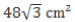are extended to form a triangle. Find the area of the triangle (in cm2).

Detailed Solution for Test: CAT Quantitative Aptitude- 6 - Question 2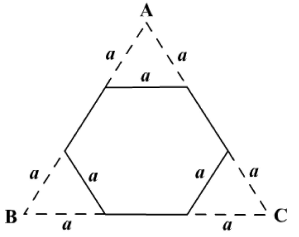As shown in the above diagram three extra equilateral triangles are formed with sides equal to the side of the hexagon.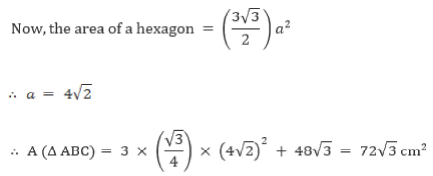Hence, option 1.

Test: CAT Quantitative Aptitude- 6 - Question 3

### Sum of the first 11 and 12 terms of an A.P. is 420 and 555 respectively. Fourth term of a G.P. (First term of the G.P. is 5) is equal to the 12th term of the A.P. What will be the common ratio of the G.P.?

Detailed Solution for Test: CAT Quantitative Aptitude- 6 - Question 3

12th term of an A.P. = Sum of first 12 terms - Sum of first 11 terms = 555 - 420= 135

4thterm of G.P. = 12th term of an A.P. =135

Let the first term and common ratio of G.P. be a and r.

4th term pf G.P. = ar3 = 135 .

∴ r = 135/5 = 27

∴ r = 3 Answer: 3

Option 3

Test: CAT Quantitative Aptitude- 6 - Question 4

In a group of six employees, an employee having a salary of Rs. 30,000 left the company and a new employee replaced him. The average salary of the six employees now decreases by Rs. 2,000. What is the salary of the new employee?

Detailed Solution for Test: CAT Quantitative Aptitude- 6 - Question 4

As average salary decreased by Rs. 2,000 for 6 members,

Total decrease in salary = 2000 x 6 = Rs. 12,000

Salary of the new employee = Salary of the person who left - total decrease in salary = 30000 - 12000 = Rs. 18,000

Hence, option 3.

Test: CAT Quantitative Aptitude- 6 - Question 5

In the land of Newtonia, car registration numbers are made of 8 digits. The registration numbers have a unique characteristic, the first digit from the left is equal to the number of 0’s, the second the number of 1 ’s, the third the number of 2’s and so on. No two cars have the same registration number, then how many cars are there in Newtonia?

Detailed Solution for Test: CAT Quantitative Aptitude- 6 - Question 5

Let the digit at the ith position from the left be f l / , l < * < 8.
It is obvious that most entries will be 0.
Suppose the digit at 8th position is non zero, then there must be at least one 7 in the number. This shows that there should be a digit which is repeated 7 times. But we have already used 2 places, so it means there will be 7 things in 6 places, which is not possible.

Similarly, we can prove a7 = 0 and a6 = 0 Now, we have three 0’s in the number placed at 6th, 7th and 8th positions.

Now, it can be proven that a5 = 1 and so there must be 4 in the number and we can easily locate it at 1st position. So a1 = 4 As ai = 4, we have to place 1 more digit ‘0’ to be placed Now that we have digit 1 at 5th position, so a2 = 1 or 2

A quick check will tell a= 2, thus a= 1 and a4 = 0

∴ There is only 1 number which is 42101000.

Hence, option 1.

Test: CAT Quantitative Aptitude- 6 - Question 6

Find the number of four digit numbers such that the number formed by tens place and ones place in that order is equal to the sum of the digits at hundreds and thousands place.

Detailed Solution for Test: CAT Quantitative Aptitude- 6 - Question 6

The digits at thousands place can be anything from 1 to 9 and the digit at hundreds place can be anything from 0 to 9.

The sum of the two digits will be either a single or double digit.

∴ The number of required numbers = 9 x 10 = 90

Hence, option 4.

Test: CAT Quantitative Aptitude- 6 - Question 7

Atul is standing at point P. P is 10 m away from a tree. Atul turns to his left and walks till point S. S is 5 m away from the tree. Baljit, who is facing the tree, also turns left from P through some angle and walks till point R. The angle traced by Baljit is twice of that by Atul. If R, S and the tree are along the same line, find the distance travelled by Baljit, if the distance between R and S is 3 m.

Detailed Solution for Test: CAT Quantitative Aptitude- 6 - Question 7

Let T be the position of tree.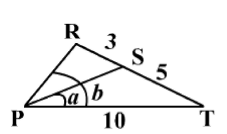m∠TPR = 2 x m∠TPS

∴ PS is the angle bisector.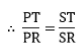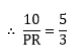∴  x = 6 meters

Hence, option 2.

Test: CAT Quantitative Aptitude- 6 - Question 8

A is standing on the foremost point of a train moving at 270 km/hr in a straight line. He starts moving in the direction opposite to that of the train at 3 m/s. At the same time B standing on the rearmost part of the train starts moving in the direction of the train at 4 m/s. A person standing outside notices that when A moves 1800 m, B is 2100 m away from A. Find the length of the train.

Detailed Solution for Test: CAT Quantitative Aptitude- 6 - Question 8

The speed of the train = 270 km/hr = 75 m/s The time after which the difference in position of A is 1800 m = 1800/(75 - 3) = 25 seconds Now, in these 25 seconds,

• A walks 25 x 3 = 75 m towards rearmost part of the train.
• B walks 25 x 4 = 100 m towards foremost point of the train.

At his stage, there is distance of 2100 m between them.

∴ The length of the train = 75 + 2100+100 = 2275 m

Hence, option 4.

Test: CAT Quantitative Aptitude- 6 - Question 9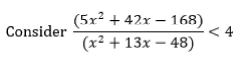Which of the following is the range of values of x?

1. -16 <x < 4

2. x > 6 orx < —16

3. 3 < x < 4

4. - 1 6 < x < 3 or 4 < x < 6

Type in the correct option number as your answer.

Detailed Solution for Test: CAT Quantitative Aptitude- 6 - Question 9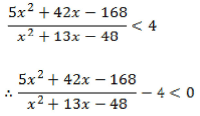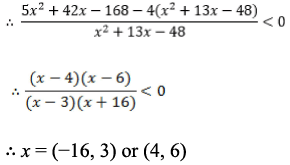Test: CAT Quantitative Aptitude- 6 - Question 10

Few students are standing one behind the other. They are asked to take chocolates from a box in sequence. Each student at even position takes as many chocolates as the total number of chocolates picked by students before him/her and each student at odd position take twice the number of chocolates taken by the student just before him/her. If the total number of chocolates picked by all the students is 512 and the first student takes as many chocolates as half the total number of student in the column then find the number of students.

Detailed Solution for Test: CAT Quantitative Aptitude- 6 - Question 10

Let the first student picks x chocolates.

∴ The total number of students = 2x

∴ The number o f chocolates tak en b y the students: x , x , 2x, 4x, 8x, 1 6 x ......
We observe that the second term onwards is a G.P.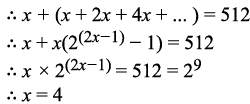Thus, the total number of students = 8

Hence, option 3.

Test: CAT Quantitative Aptitude- 6 - Question 11

A whole saler buys 100 watches from on estate at the rate of Rs.45.45 per watch and sells the mall in his own state for Rs 6817.5 He pays a certain percentage of the cost price as the tax. If he earns a total of 20% by selling the watches, then find the tax percentage levied over the purchased price.

Detailed Solution for Test: CAT Quantitative Aptitude- 6 - Question 11

Total purchase price = 45.45 x 100 = Rs. 4545 Let the tax paid be Rs. x

∴ (6817.5 - 4545 - x)/(4545 + x) = 0.2

∴ 2272.5 - x = 909 + 0.2x

∴ x = 1136.25
The tax percentage levied over cost price = (1136.25/4545) x 100 = 25%

Test: CAT Quantitative Aptitude- 6 - Question 12

What will be the remainder when 3136 is divided by 12 ?

Detailed Solution for Test: CAT Quantitative Aptitude- 6 - Question 12

When 31,32, 33 and 34 divided by 12 leaves remainder 3, 9, 3 and 9 respectively.

As the pattern repeats itself after 2 steps, 3 odd number leaves remainder 3 and 3even number leaves remainder 9.

∴ R em ainder of 3136 when divided by 12 = 9

Hence, option 3.

Test: CAT Quantitative Aptitude- 6 - Question 13

20 Chocolates are to be distributed among 5 children. In how many ways can this be done if the children get only an even number of chocolates?

Detailed Solution for Test: CAT Quantitative Aptitude- 6 - Question 13

Let the chocolates received by the five children be a, b, c, d, and e respectively.

∴ a+b+c+d+e = 20

Since only even number of chocolates are allowed we can make the following substitution,

2 (p+q+r+s+t) = 20

∴ P+q+r+s+t = 10

Now, no student can get zero chocolates.

Thus, the total number of ways =

(n-1)C(r-1)

Here, n = 10 and r = 5

∴ The total number of ways = 9C4 = 126

Hence, option 1.

Test: CAT Quantitative Aptitude- 6 - Question 14

A bank pays 4.5% compound interest per month for a principal amount P.
The amount becomes Pm in m months. Which of the following options best represent the graph between Pm (y-axis) and m (x-axis) will be:

Detailed Solution for Test: CAT Quantitative Aptitude- 6 - Question 14

From the given conditions;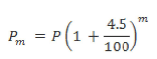We can see that Pm increases exponentially as m increases.

Option 3 is the only possible answer.

Hence, option 3.

Test: CAT Quantitative Aptitude- 6 - Question 15

Let g(x +1) g(x - 1) = g(x). then for what integral values of p would we have g(x+p) = g(x)?

Detailed Solution for Test: CAT Quantitative Aptitude- 6 - Question 15

Let g(x) = a and g(x - 1 ) = b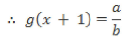Let x = x + 1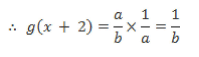Similarly, g(x + 3) = l/a, g(x + 4) = bla, g(x + 5) = b, g(x + 6) = a .. .and so on.
∴ g(x + 6) = g(x)

Answer: 6 optin 3

Test: CAT Quantitative Aptitude- 6 - Question 16

ABCD is a rectangle. ΔAED is inscribed in it as shown in the diagram. Find the area of ABCD.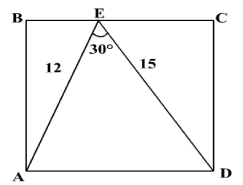Detailed Solution for Test: CAT Quantitative Aptitude- 6 - Question 16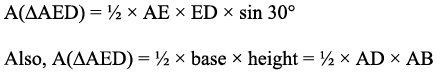Now, AD x AB is the area of the rectangle.

∴ Area of rectangle = AD x AB = AE x ED x sin 30° = 90

Option 2

Test: CAT Quantitative Aptitude- 6 - Question 17

A petrol distributor adulterates petrol with kerosene and naphthalene. There are two containers of different capacities which contain this adulterated petrol. Container 1 contains 30% petrol and container 2 contains 45% petrol. If both these mixtures are mixed in a larger container in such a ratio that kerosene in it is 25% and naphthalene is 40%. Find the capacity of container 2(in litre) if the capacity of container 1 is 8 litres.

Detailed Solution for Test: CAT Quantitative Aptitude- 6 - Question 17

The correct option is Option B.

Let the capacity of Container 1 be ‘a’ litres and that of Container 2 be ‘6’ litres.

∴ Petrol in container 1 = 0.3a

Petrol in container 2 = 0.456

Similarly, petrol in the new container = 0.35(a + b)

∴ 035 a + 0.45 b = 0.35(a + b)

∴ a = 2b

Since a = 8, b = 4.

Hence, the capacity of container 2 is 8 litres.

Hence, the answer is 4

Test: CAT Quantitative Aptitude- 6 - Question 18

In the equation x2 + 17x - p = 0, difference between the sum and the product of the roots is 4 more than the sum itself. What is the value of p ?

Detailed Solution for Test: CAT Quantitative Aptitude- 6 - Question 18

Let sum and product of the roots be s and p' respectively. s = -17 and p' = —p

|s - p' | = s + 4
| - 17 +p | = - 17 + 4
∴ p = 4 or p = 30

Hence, option 4.

Test: CAT Quantitative Aptitude- 6 - Question 19

A bucket can be filled by two taps independently in 10 minutes and 15 minutes respectively. But due to some obstruction present in both the taps, the former delivers water at 2/5th of its capacity and the latter at 3/5th of its capacity. But for a while the obstruction got removed and the bucket gets filled in 3 minutes from the moment. For how long did the tap deliver water with obstructions present in them?

Detailed Solution for Test: CAT Quantitative Aptitude- 6 - Question 19

When the taps are working at 2/5th and 3/5th of their capacity, they will take 10 x 5/2 = 25 minutes and 15 x 5/3 = 25 minutes respectively.
Let the obstruction be for time 't' minutes.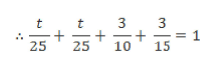∴ t = 6.25 minutes

Hence, option 4.

Test: CAT Quantitative Aptitude- 6 - Question 20

A square cuts the x m d y axes at (2, 0); (-2, 0) and (0, 2);(0, -2). I f the axes are rotated by 60° in anticlockwise direction, then find the ratio (smaller to larger) in which each side of the square will be divided into by any of the axes.

Detailed Solution for Test: CAT Quantitative Aptitude- 6 - Question 20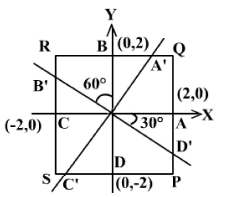As shown in the figure the square PQRS cuts the axes at A(2,0), B(0,2), C (-2,0) and D(0, - 2).
When rotated by 60° the axes will cut the square at A', B', C' and D' respectively.

PD’ = AP - AD'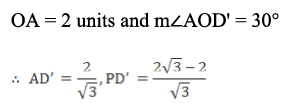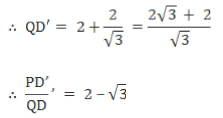Hence, option 3.

Test: CAT Quantitative Aptitude- 6 - Question 21

The sum of n terms of an A.P. is given by (5n2 + 7n + 4)/4. What will be the fifth term of this A.P?

Detailed Solution for Test: CAT Quantitative Aptitude- 6 - Question 21

Let sum of 4 terms and sum of 5 terms be S4 and S5 respectively.
S5 = [5(5)2 + 7(5) + 4]/4 = 41

S4 = [5(4)2 + 7(4) + 4]/4 = 28

5th term of an A.P. = S5 - S4 = 13

Hence, option 2.

Test: CAT Quantitative Aptitude- 6 - Question 22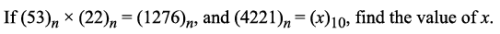Detailed Solution for Test: CAT Quantitative Aptitude- 6 - Question 22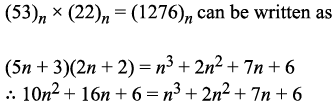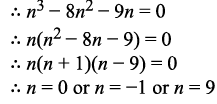But n has to be a natural number.

∴ n = 9 Now,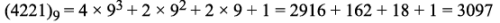Option 2

Test: CAT Quantitative Aptitude- 6 - Question 23

The average age of a village X at the start of the year 2008 is 30 years. Every year starting from 2009, migrants - amounting to 20% of the population of village X in 2008 - move to village X. The average age at entry, for every batch of migrants is 5 years less than that of the village X at the start of 2008. Find the average age of the village X just after the third batch of migrants enter it.

Detailed Solution for Test: CAT Quantitative Aptitude- 6 - Question 23

Let the current population of the village be x.

The population of the village just after the third batch of migrants entre village X = x + 3(0.2x) = 1.6x

The total sum of the ages of the migrants just after the third batch of migrants entre village X = 0.2x x 27 + 0.2x x 26 + 0.2x x 25 = 15.6x

The average age of the village after 3 years = (33x + 15.6x )/1.6x = 30.375 years

Hence, option 4.

Test: CAT Quantitative Aptitude- 6 - Question 24

Roshan has three daughters Riya, Piya and Siya. He divides 21 chocolates among them such that for every chocolate Riya receives, Siya receives 3 chocolates and for every chocolate that Piya receives, Riya receives 5 chocolates. How many chocolates did Siya get?

Detailed Solution for Test: CAT Quantitative Aptitude- 6 - Question 24

The correct option is Option D.

The number of chocolates received by Siya is multiple of 3.

Among the given options, only 15 is multiple of 3.

So, Siya gets 15 chocolates.

Test: CAT Quantitative Aptitude- 6 - Question 25

Two explorers are at the North and South pole of the Earth respectively. They use a revolutionary new supeijet to travel towards each other, both travelling along the same meridian of longitude. The speed of the one at the North pole initially is 1884 km/hr, and the speed of the other is 1256 km/hr. They meet in one-fourth of a day. What is the approximate diameter of the Earth (in kilometers)?

Detailed Solution for Test: CAT Quantitative Aptitude- 6 - Question 25

Let the radius of the Earth be r.
The relative speed at which the explorers are travelling towards each other = 1884+ 1256 = 3140 km/hr

∴ This is approximately IOOOπ km/hr.
The time taken for the meeting is one-fourth of a day, i.e. 6 hours.

They travel a total distance of 6OOOπ km to meet each other.

Now, this distance is approximately half the circumference of the Earth, which is equal to (πr).

∴ r = 6000 km

∴ Diameter of the Earth = 2r= 12000 km

Hence, option 4.

Test: CAT Quantitative Aptitude- 6 - Question 26

The energy (E) stored in a capacitor is directly proportional to the square of the charge (Q) and inversely proportional to the capacitance (Cp). When the charge was 10C and capacitance was 0.1 F, the energy stored was 500 J. What is the charge on a capacitor of five times the storage capacity of the former if its capacitance is 320 mF? [Assume that if the energy stored is measured in Joules (J) then the corresponding units for measurement of charge and capacitance are Coulombs (C) and Farads (F).]

Detailed Solution for Test: CAT Quantitative Aptitude- 6 - Question 26

Energy (E) stored in a capacitor is directly proportional to the square of the charge ( 0 and inversely proportional to the capacitance Cp.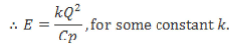We know that, E = 500 J if Q= 10C and C= 0.1 F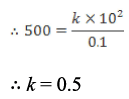In the second case we know, E = 2500 J (The storage capacity of this capacitor is 5 times the previous one) and Cp = 320 mF = 0.32 F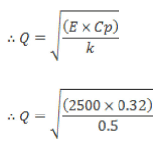∴ Q = 40 C
Hence, option 2.

Test: CAT Quantitative Aptitude- 6 - Question 27

Two smaller sides of a right-angled triangle are 15 cm and 20 cm. What is the in-radius of the triangle (in cm)?

Detailed Solution for Test: CAT Quantitative Aptitude- 6 - Question 27

Inradius of right-angled triangle = (Sum of the perpendicular sides - Hypotenuse)/2 By Pythagoras theorem,

Hypotenuse = √(152 + 202) = 25 cm Inradius = (15 + 20 - 25)/2 = 5 cm Answer: 5

Hence Option 3

Test: CAT Quantitative Aptitude- 6 - Question 28

What is the difference between the maximum and minimum value that f(x) can take?

Detailed Solution for Test: CAT Quantitative Aptitude- 6 - Question 28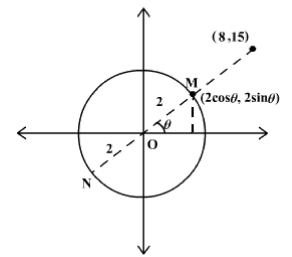Consider a point (a, b) at a distance of 2 units from the origin such that the line joining the origin and (a, b) makes an angle of 6 with the positive direction of the x-axis.
Then from the above figure we can see that a = 2cos θ, and b = 2sin θ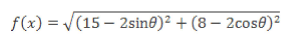Thus,f(x) is nothing but the distance between (8, 15) and (a, b).
From the figure, we can easily see that this distance will be 1.1. Maximised when (a, b) is at point N and lies on the line joining (8, 15) and the origin.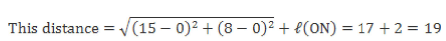2. Minimised when {a, b) is at point M and lies on the line joining (8, 15) and the origin.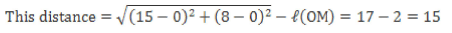Thus, the difference between the maximum and minimum value that f(x) can take is 19 — 15 = 4.
Hence, option 1.

Test: CAT Quantitative Aptitude- 6 - Question 29

f(x + y) = f(x) x f(y) for all x, y > 0. If/(1) = 2, then what is the value of f(2) +f(3) + f(4 ) ?

Detailed Solution for Test: CAT Quantitative Aptitude- 6 - Question 29

We have,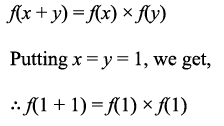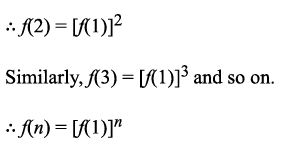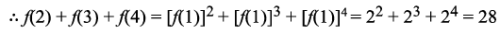Hence Option 2

Test: CAT Quantitative Aptitude- 6 - Question 30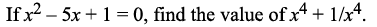Detailed Solution for Test: CAT Quantitative Aptitude- 6 - Question 30

Since the value of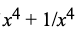is to be found, first try and express the given equation in terms of x + l/x.
To do so, divide the given equation by x on both sides.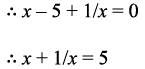Squaring both sides,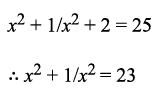Again squaring both sides,

x4+1/x4 + 2 = 529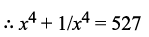Hence Option 3

Test: CAT Quantitative Aptitude- 6 - Question 31

In the given figure, a semicircle with centre O has radius 1 cm and ΔAOB is an equilateral triangle. Find the area of the shaded region (in cm2).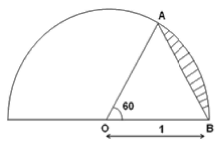Detailed Solution for Test: CAT Quantitative Aptitude- 6 - Question 31

Area of the triangle =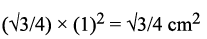Area of sector AOB = (60/360) x (22/7) x (1)2 = 11/21 cm2

Area of the shaded region = (11/21) - (√3/4) cm

Hence, option 1.

Test: CAT Quantitative Aptitude- 6 - Question 32

The ratio of the area of two similar right angled triangle is 4 : 9. Find the ratio of the volume of the cones formed by rotating the triangles about their hypotenuse.

Detailed Solution for Test: CAT Quantitative Aptitude- 6 - Question 32

Area of the triangle = (1/2) x b x h

Since, the two triangles are similar the corresponding sides will be in the same ratio

i.e if b, h and H are the base, height and hypotenuse of one of the triangles and b, h ’ and H' of the other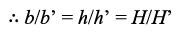Since, the area o f the triangles is in the ratio 4 : 9 .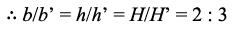Also, the radius of the cones will be in the same ratio.
Therefore, the required ratio = 8 : 2 7 Hence, option 2.

Test: CAT Quantitative Aptitude- 6 - Question 33

If a + b + c = 0, A = a3+b3+c3 and B = 3 abc, then find the value of logA(B2)

Detailed Solution for Test: CAT Quantitative Aptitude- 6 - Question 33

We know that when, a + b + c = 0 ; a3 + b3 + c3 = 3 abc
A = B
Now,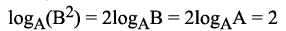Hence, option 3.

Test: CAT Quantitative Aptitude- 6 - Question 34

The area of the incircle of an equilateral triangle is (100/9)π cm2. Find the area of the largest circle that can be accommodated in the rest of the space the triangle touching two sides.

Detailed Solution for Test: CAT Quantitative Aptitude- 6 - Question 34

For an equilateral triangle the centroid and incentre are the same.
So, the radius of the circle will be one-third of the height.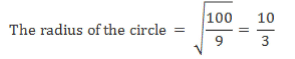The required circle will be the incircle of the triangle formed by the two sides and tangent to the incircle.

The height of this triangle = 10/3

The area of the required incircle = (100/81)π cm2

Hence, option 1.

## CAT Mock Test Series

2 videos|24 docs|89 tests
 Use Code STAYHOME200 and get INR 200 additional OFF Use Coupon Code
Information about Test: CAT Quantitative Aptitude- 6 Page
In this test you can find the Exam questions for Test: CAT Quantitative Aptitude- 6 solved & explained in the simplest way possible. Besides giving Questions and answers for Test: CAT Quantitative Aptitude- 6, EduRev gives you an ample number of Online tests for practice

## CAT Mock Test Series

2 videos|24 docs|89 tests

### How to Prepare for CAT

Read our guide to prepare for CAT which is created by Toppers & the best Teachers